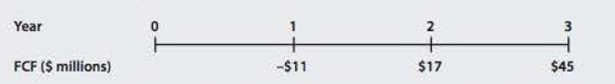Chapter 9, Problem 15PFundamentals of Financial Manageme...

15th Edition
Eugene F. Brigham + 1 other
ISBN: 9781337395250

Solutions

Chapter
SectionFundamentals of Financial Manageme...

15th Edition
Eugene F. Brigham + 1 other
ISBN: 9781337395250
Textbook Problem

CORPORATE VALUATION Dantzler Corporation is a fast-growing supplier of office products. Analysts project the following free cash flows (FCFs) during the next 3 years, after which FCF is expected to grow at a constant 5% rate. Dantzler’s WACC is 11%.a. What is Dantzler’s horizon, or continuing, value? (Flint: Find the value of all free cash flows beyond Year 3 discounted back to Year 3.) b. What is the firm’s value today? c. Suppose Dantzler has $112.60 million of debt and 25 million shares of stock outstanding. What is your estimate of the current price per share? a. Summary Introduction To compute: The horizon value for Company D. Introduction: Corporate Valuation: The process to evaluate a firm on the basis of its future operations and the results of those operations is called corporate valuation. It is a useful tool to analyze a firm’s stock for investment purposes. Horizon Value: The value at a specified future date that includes the total of discounted amount to be received after that future date is called as the horizon value. The future date that is considered here, is the one after which the growth in cash flows will be constant forever. Explanation Given information: Free cash flow at the end of third year is$45 million.

Constant growth rate is 5% or 0.05.

WACC is 11% or 0.11.

Formula to compute the horizon value,

Horizon value=FCF3(1+g)WACCg

Where,

• FCF3 is the free cash flow at the end of 3rd year.
• g is the constant growth rate of free cash flow.
• WACC is the weighted average cost of capital.

Substitute \$45 million for FCF3 , 0

b.

Summary Introduction

To compute: The firm’s value for Company D.

Introduction:

Firm’s Value:

The firm’s value is also known as the intrinsic value, it is the total of all present values of future free cash flows a company estimates from its operations. This is used as per the corporate valuation model to evaluate a firm.

c.

Summary Introduction

To compute: The current share price of Company D.

Still sussing out bartleby?

Check out a sample textbook solution.

See a sample solution

The Solution to Your Study Problems

Bartleby provides explanations to thousands of textbook problems written by our experts, many with advanced degrees!

Get Started

What are the advantages of a corporation?

Foundations of Business (MindTap Course List)

Calculate the missing items in the following:

College Accounting (Book Only): A Career Approach

Suppose a firm makes the following policy changes. If the change means that external nonspontaneous financial r...

Fundamentals of Financial Management, Concise Edition (with Thomson ONE - Business School Edition, 1 term (6 months) Printed Access Card) (MindTap Course List)